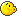## Problem J4: Global Warming

Your task is to help scientists predict the trend of the global warming. One of the hypotheses they are considering is that over long periods of time, the average temperature follows certain cycles, but each time the cycle starts from a higher temperature level. The temperatures are measured over five-year averages, and expressed in tenths of a degree.

For example, if the following five-year averages are observed:

3, 4, 6, 4, 5, 7, 5

then we can calculate that the temperature changes first 1 tenth up, then 2 up, then 2 down, 1 up, 2 up, and 2 down. There is a cycle of changes of length three which covers all of the temperature differences: (+1, +2, −2). In other words, if we look at the differences starting at the first position, there is a cycle of length three of the form (+1, +2, −2) followed by another cycle of length three of exactly the same form.

By way of another example, suppose the following average temperatures are observed:

3, 4, 6, 7

In this case, there is a difference of one up, two up, then one up. We would consider the shortest cycle to be length two in this case: the cycle (+1, +2). Notice that this cycle occurs once, followed by one truncated occurrence of exactly the same cycle.

Your task is to find the shortest such cycle from a given sequence of temperatures.

### Input Format

The input consists of a number of test cases. Each test case starts with the number n (1 ≤ n ≤ 20), representing the number of temperatures in a sequence, followed by the sequence of n temperatures. You may assume that each temperature input is an integer in the range −1000…1000, inclusive. The numbers are separated by a single space. The last test case is indicated by a zero and should produce no output.

### Output Format

For each test case, produce the length of the shortest temperature cycle. The cycle always exists, since the whole sequence could be treated as one long cycle.

### Sample Input

```7 3 4 6 4 5 7 5
3 1 3 5
3 1 4 5
4 3 4 6 7
0```

### Sample Output

```3
1
2
2```

Point Value: 10 (partial)
Time Limit: 2.00s
Memory Limit: 16M

Languages Allowed:
C++03, PAS, C, HASK, ASM, RUBY, PYTH2, JAVA, PHP, SCM, CAML, PERL, C#, C++11, PYTH3

• (0/0)
I believe I got the algorithm but for some reason the last case doesn't work for me

I checked with the solutions and the test cases is:

18 -10 1 3 4 6 7 9 10 12 13 15 16 18 19 21 22 24 25
0

So I think there must be some kind of mistake?

• (0/0)
you are outputting 2 in your program tho....

• (0/0)• (2/1)
For some reason, I am only getting 3/15 even though my submission worked with all test cases. Can someone please tell me where the error is?

• (0/0)
My program works with the sample test cases, however, it received a zero score on the test cases from the judge.

• (1/0)
You're getting this on every run:
``Exception_in_thread "main" java.lang.NegativeArraySizeException    at ccc10j4.main(ccc10j4.java:82)``

• (1/0)